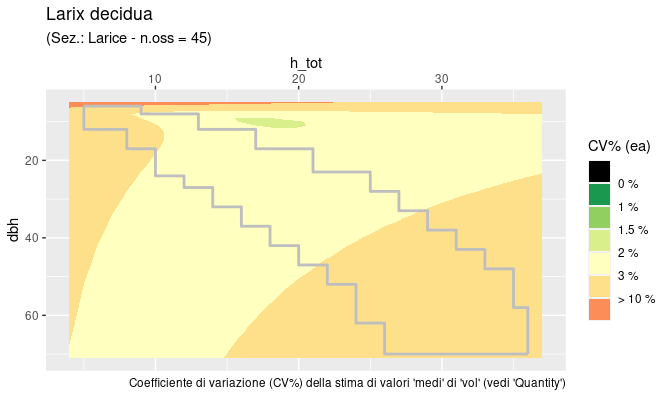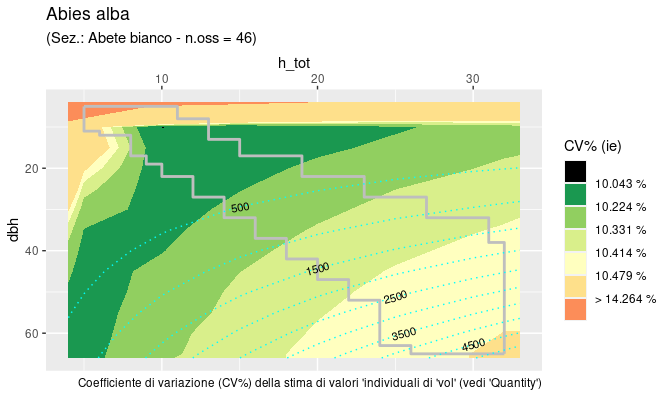# ForITThe functions estimating tree volume and aboveground (ABG) phytomass for the 2nd Italian National Forest Inventory (2005) are documented in Tabacchi et al. (2011). (The section of the the publication exposing “Methods and results global evaluation” is accessible here Regression models have been developed based on sample trees collected within three successive projects, in order to consider, as much as possible, all of the most relevant tree species present in Italian forests.

Providing such a general and uniform estimation tool, these functions have been adopted by Italian foresters as reference functions. Many professionals and researchers have copied, from the paper print or the PDF file, the small subset of coefficient values required to tackle their problem at hand.
The package offers an easier (and more reliable) way to proceed. Moreover, since coefficients were not readily available through a digital tool, the valuable information that the work developed for the evaluation of estimates accuracy, has been practically ignored.
Using the ForIT package, all estimates are associated with accuracy evaluations, through attributes.

The independent variables used in the model are the diameter-at-breast-height ($$dbh$$) and the total tree height ($$h_{tot}$$). The general model formulation adopted is the following:

{y_0 = b_0 + b_1 × dbh^2 × h_{tot} + b_2 × dbh}

where $$y_0$$ is the volume or AGB phytomass.

Through the ForIT package, all the Tabacchi et al. (2011) volume and phytomass estimation functions are readily available in the R environment.

### Package installation

The version under development can be installed using:

devtools::install_gitlab("NuoroForestrySchool/forit")  

### Detailed description with examples

library(tidyverse)
library(ForIT)

The package functions cover three main areas:
1) the estimation of tree quantities, for single trees or as totals;
2) tabulation, to obtain the volume or phytomass tables (INFCtabulate());
3) graphical accuracy assessment (INFCaccuracyPlot0() and INFCaccuracyPlot()).

Connecting the package with well established operational standards, the ‘tree species codes’ used by ForIT are the “EPPO codes”. (See https://gd.eppo.int, for more info on EPPO project and data(INFCspecies) for a complete list of species used in this package).

#### 1) Volume and AGB tree estimates

Single tree estimates are obtained using the INFCvpe() function.
Otherwise, if interested in estimates for groups of trees, one can refer to the INFCvpeSUM family of functions.

##### 1.1 - Single tree estimates

Estimation of the volume of one Acer campestre tree (EPPO code is ‘ACRCA’) with diameter-at-breast-height equal to 22 cm and 14 m height.

vol <- INFCvpe("ACRCA", dbh.cm = 22, htot.m = 14)

The default estimated quantity is volume (quantity = "vol"). In this specific case, the estimated volume is 252.96 dm3.

> round(as.numeric(vol),2)
#  252.96

The codes and definitions of all the quantities the functions estimate are listed in the ‘Quantities’ table:

> ForIT::Quantities
# # A tibble: 5 × 2
#   quantity quantity_definition
#    <chr>    <chr>
#  1 vol      volume of the stem and large branches [dm^3]
#  2 dw1      phytomass of the stem and large braches [kg]
#  3 dw2      phytomass of the small branches [kg]
#  4 dw3      phytomass of the stump [kg]
#  5 dw4      phytomass of the whole tree [kg]   

Volume is expressed in [dm3], while all the quantities referred to phytomass are expressed in [kg].

The attributes accompanying each estimated value, specify accuracy evaluations for the selected quantity. wrv is the ; Var_ea is the variance for an estimated average;
Var_ie the variance for an individual estimate;
InDomain is a logical indicating whether the (dbh, htot) point lies out of the domain explored by the experimental data (see INFCtabulate()).

> vol
#  252.9581
# attr(,"pag")       # page number, referred to original source
#  231
# attr(,"quantity")  # see above
#  "vol"
# attr(,"wrv")       # weighted residual variance
#  2.271e-05
# attr(,"Var_ea")    # variance for an estimated average
#  33.17182
# attr(,"Var_ie")    # variance for an individual estimate
#  1075.883
# attr(,"InDomain")  # logical indicating whether the (dbh, htot) point lies within the domain
#  TRUE
##### 1.2 - Estimates for groups of trees

Cumulative estimation of the volume or phytomass of groups of trees is just the summation of the values computed with INFCvpe(), but the computation of accuracy estimates is improved using these summation functions.

Two approaches are available.

##### 1.2.1 - Via INFCvpe_summarise()

This function, alternative to dplyr::summarise(), returns a dataframe (tibble) with unique values combinations in the grouping column/s, defined via group_by(), plus a fixed set of columns for estimates and accuracy evaluation.

ForIT_test_data %>%
INFCvpe_summarise("specie", "d130", "h_dendro")
# A tibble: 1 × 6
# Groups:   quantity 
#     quantity     n n_out      est   cihw     p
#     <chr>    <int> <int>    <dbl>  <dbl> <dbl>
#   1 vol         17     1    10335.  927.  0.95
##### 1.2.2 - Within dplyr::summarise()

for each set of rows, defined via group_by(), following aggregation functions are available: * INFCvpe_sum(), that returns the sum of the estimated quantities; * INFCvpe_ConfInt(), that returns ‘confidence interval half width’; * INFCvpe_OutOfDomain(), that returns the number of ‘out of domain’ (dbh, h_tot) pairs included in the summation.

For more details on the functions of this family: ?ForIT::INFCvpeSUM

#### 2) INFCtabulate

Volume and phytomass functions are tabulated in Tabacchi et al. (2011a). Printed numbers serve as reference to verify that coded functions return expected results and, more specifically, empty spaces (or NA) in the printed tables limit function applicability domain. In other words, measurement data used to estimate function coefficient values, cover only the portion of the (dbh, htot) plane where numbers are printed.

> INFCtabulate("ACRCA")
# EPPO code:  ACRCA  - pag =  231  - quantity = ' vol '
# htot.m
# dbh.cm    5    8    11    14    17     20     23
# 5       6.3  9.1    NA    NA    NA     NA     NA
# 10     20.2 31.4  42.5    NA    NA     NA     NA
# 15       NA 68.4  93.5 118.5    NA     NA     NA
# 20       NA   NA 164.9 209.3 253.8  298.3     NA
# 25       NA   NA    NA 326.2 395.7  465.2     NA
# 30       NA   NA    NA 468.9 569.0  669.2  769.3
# 35       NA   NA    NA    NA 773.9  910.2 1046.5
# 40       NA   NA    NA    NA    NA 1188.3 1366.3
# ---

More info at ?ForIT::INFCtabulate.

#### 3) Graphical evaluation of estimates accuracy and reliability

The tabulation described in §2 covers a limited region of the dbh by h_tot rectangle.
This region is the “domain” of the reliable estimates, based on the distribution of the sample trees used to calibrate the functions. The coefficient of variation (CV = standard_deviation / estimate) is computed and plotted (as ‘filled contours’) for the whole rectangular area, the limits of the region of reliable estimates (the “domain”), is superimposed as a light coloured line. Function output is a ggplot object that can be used by itself or as a background on top of which the user can plot his/her data to verify eventual accuracy or reliability problems.

##### Two functions are available.

INFCaccuracyPlot0() - produces, much faster, the plots at the finest resolution, using pre-calculated values stored in a specific auxiliary dataframe (see INFC_CVgrid), necessarily leaving less customization freedom.

INFCaccuracyPlot0("LAXDE")INFCaccuracyPlot() - allows the plots to be fully customized but, beware, all values required for the ‘fill’ will be computed and, at finer resolution, the process can be slow.

INFCaccuracyPlot("ABIAL", ie.Var = T, plot.est = T, cv.ul = .15, fixed = F)Gasparini, P., Tabacchi, G. (eds), 2011. L’Inventario Nazionale delle Foreste e dei serbatoi forestali di Carbonio INFC 2005. Secondo inventario forestale nazionale italiano. Metodi e risultati. Edagricole. 653 pp.

Tabacchi G., Di Cosmo L., Gasparini P., Morelli S., 2011. Stima del volume e della fitomassa delle principali specie forestali italiane. Equazioni di previsione, tavole del volume e tavole della fitomassa arborea epigea. Stima del volume e della fitomassa delle principali specie forestali italiane. Equazioni di previsione, tavole del volume e tavole della fitomassa arborea epigea. 412 pp. full text

Tabacchi G., Di Cosmo L., Gasparini P., 2011. Aboveground tree volume and phytomass prediction equations for forest species in Italy. European Journal of Forest Research 130: 6 911-934.